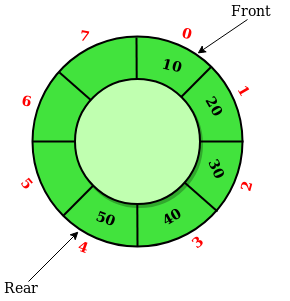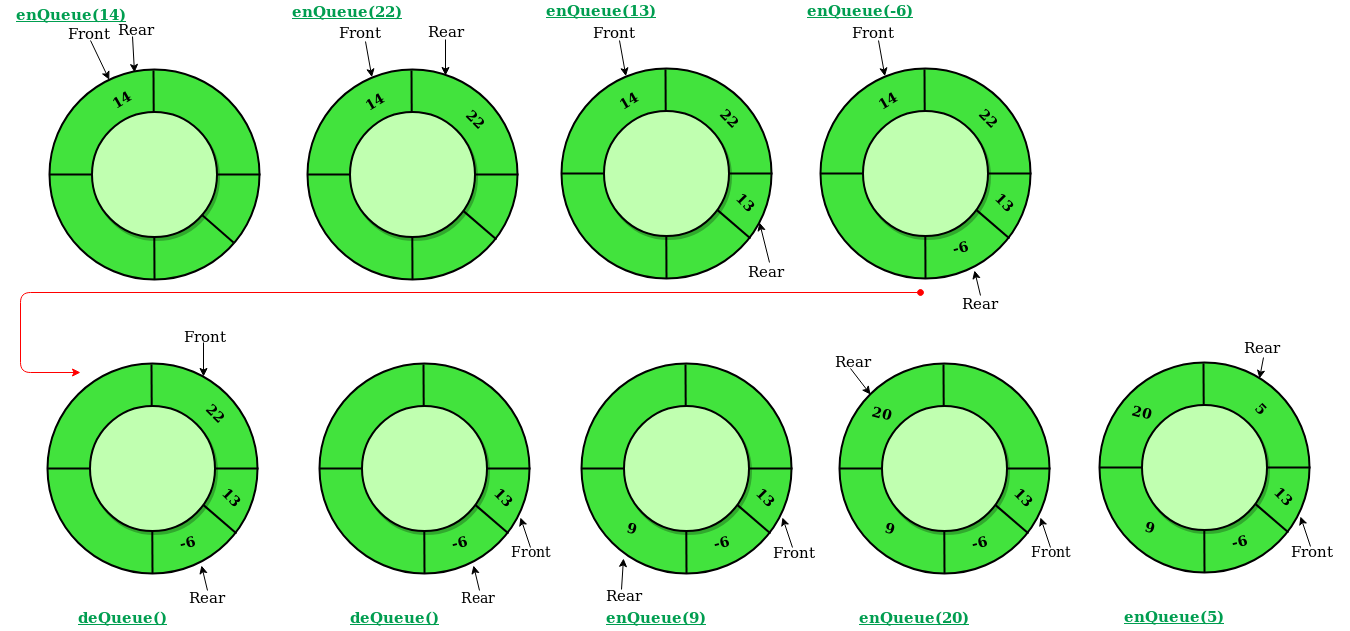GeeksforGeeks App
Open AppBrowser
Continue

# Introduction to Circular Queue

## What is a Circular Queue?

A Circular Queue is an extended version of a normal queue where the last element of the queue is connected to the first element of the queue forming a circle.

The operations are performed based on FIFO (First In First Out) principle. It is also called ‘Ring Buffer’In a normal Queue, we can insert elements until queue becomes full. But once queue becomes full, we can not insert the next element even if there is a space in front of queue.

## Operations on Circular Queue:

• Front: Get the front item from the queue.
• Rear: Get the last item from the queue.
• enQueue(value) This function is used to insert an element into the circular queue. In a circular queue, the new element is always inserted at the rear position.
• Check whether the queue is full – [i.e., the rear end is in just before the front end in a circular manner].
• If it is full then display Queue is full.
• If the queue is not full then,  insert an element at the end of the queue.
• deQueue() This function is used to delete an element from the circular queue. In a circular queue, the element is always deleted from the front position.
• Check whether the queue is Empty.
• If it is empty then display Queue is empty.
• If the queue is not empty, then get the last element and remove it from the queue.

### Illustration of Circular Queue Operations:

Follow the below image for a better understanding of the enqueue and dequeue operations.Working of Circular queue operations

## How to Implement a Circular Queue?

A circular queue can be implemented using two data structures:

Here we have shown the implementation of a circular queue using an array data structure.

## Implement Circular Queue using Array:

1. Initialize an array queue of size n, where n is the maximum number of elements that the queue can hold.
2. Initialize two variables front and rear to -1.
3. Enqueue: To enqueue an element x into the queue, do the following:
• Increment rear by 1.
• If rear is equal to n, set rear to 0.
• If front is -1, set front to 0.
• Set queue[rear] to x.
4. Dequeue: To dequeue an element from the queue, do the following:
• Check if the queue is empty by checking if front is -1.
• If it is, return an error message indicating that the queue is empty.
• Set x to queue[front].
• If front is equal to rear, set front and rear to -1.
• Otherwise, increment front by 1 and if front is equal to n, set front to 0.
• Return x.

Below is the implementation of the above idea:

## C++

 `// C or C++ program for insertion and``// deletion in Circular Queue``#include``using` `namespace` `std;`` ` `class` `Queue``{``    ``// Initialize front and rear``    ``int` `rear, front;`` ` `    ``// Circular Queue``    ``int` `size;``    ``int` `*arr;``public``:``    ``Queue(``int` `s)``    ``{``       ``front = rear = -1;``       ``size = s;``       ``arr = ``new` `int``[s];``    ``}`` ` `    ``void` `enQueue(``int` `value);``    ``int` `deQueue();``    ``void` `displayQueue();``};`` ` ` ` `/* Function to create Circular queue */``void` `Queue::enQueue(``int` `value)``{``    ``if` `((front == 0 && rear == size-1) ||``            ``(rear == (front-1)%(size-1)))``    ``{``        ``printf``(``"\nQueue is Full"``);``        ``return``;``    ``}`` ` `    ``else` `if` `(front == -1) ``/* Insert First Element */``    ``{``        ``front = rear = 0;``        ``arr[rear] = value;``    ``}`` ` `    ``else` `if` `(rear == size-1 && front != 0)``    ``{``        ``rear = 0;``        ``arr[rear] = value;``    ``}`` ` `    ``else``    ``{``        ``rear++;``        ``arr[rear] = value;``    ``}``}`` ` `// Function to delete element from Circular Queue``int` `Queue::deQueue()``{``    ``if` `(front == -1)``    ``{``        ``printf``(``"\nQueue is Empty"``);``        ``return` `INT_MIN;``    ``}`` ` `    ``int` `data = arr[front];``    ``arr[front] = -1;``    ``if` `(front == rear)``    ``{``        ``front = -1;``        ``rear = -1;``    ``}``    ``else` `if` `(front == size-1)``        ``front = 0;``    ``else``        ``front++;`` ` `    ``return` `data;``}`` ` `// Function displaying the elements``// of Circular Queue``void` `Queue::displayQueue()``{``    ``if` `(front == -1)``    ``{``        ``printf``(``"\nQueue is Empty"``);``        ``return``;``    ``}``    ``printf``(``"\nElements in Circular Queue are: "``);``    ``if` `(rear >= front)``    ``{``        ``for` `(``int` `i = front; i <= rear; i++)``            ``printf``(``"%d "``,arr[i]);``    ``}``    ``else``    ``{``        ``for` `(``int` `i = front; i < size; i++)``            ``printf``(``"%d "``, arr[i]);`` ` `        ``for` `(``int` `i = 0; i <= rear; i++)``            ``printf``(``"%d "``, arr[i]);``    ``}``}`` ` `/* Driver of the program */``int` `main()``{``    ``Queue q(5);`` ` `    ``// Inserting elements in Circular Queue``    ``q.enQueue(14);``    ``q.enQueue(22);``    ``q.enQueue(13);``    ``q.enQueue(-6);`` ` `    ``// Display elements present in Circular Queue``    ``q.displayQueue();`` ` `    ``// Deleting elements from Circular Queue``    ``printf``(``"\nDeleted value = %d"``, q.deQueue());``    ``printf``(``"\nDeleted value = %d"``, q.deQueue());`` ` `    ``q.displayQueue();`` ` `    ``q.enQueue(9);``    ``q.enQueue(20);``    ``q.enQueue(5);`` ` `    ``q.displayQueue();`` ` `    ``q.enQueue(20);``    ``return` `0;``}`

## Java

 `// Java program for insertion and``// deletion in Circular Queue``import` `java.util.ArrayList;`` ` `class` `CircularQueue{`` ` `// Declaring the class variables.``private` `int` `size, front, rear;`` ` `// Declaring array list of integer type.``private` `ArrayList queue = ``new` `ArrayList();`` ` `// Constructor``CircularQueue(``int` `size)``{``    ``this``.size = size;``    ``this``.front = ``this``.rear = -``1``;``}`` ` `// Method to insert a new element in the queue.``public` `void` `enQueue(``int` `data)``{``     ` `    ``// Condition if queue is full.``    ``if``((front == ``0` `&& rear == size - ``1``) ||``      ``(rear == (front - ``1``) % (size - ``1``)))``    ``{``        ``System.out.print(``"Queue is Full"``);``    ``}`` ` `    ``// condition for empty queue.``    ``else` `if``(front == -``1``)``    ``{``        ``front = ``0``;``        ``rear = ``0``;``        ``queue.add(rear, data);``    ``}`` ` `    ``else` `if``(rear == size - ``1` `&& front != ``0``)``    ``{``        ``rear = ``0``;``        ``queue.set(rear, data);``    ``}`` ` `    ``else``    ``{``        ``rear = (rear + ``1``);``     ` `        ``// Adding a new element if ``        ``if``(front <= rear)``        ``{``            ``queue.add(rear, data);``        ``}``     ` `        ``// Else updating old value``        ``else``        ``{``            ``queue.set(rear, data);``        ``}``    ``}``}`` ` `// Function to dequeue an element``// form th queue.``public` `int` `deQueue()``{``    ``int` `temp;`` ` `    ``// Condition for empty queue.``    ``if``(front == -``1``)``    ``{``        ``System.out.print(``"Queue is Empty"``);``         ` `        ``// Return -1 in case of empty queue``        ``return` `-``1``; ``    ``}`` ` `    ``temp = queue.get(front);`` ` `    ``// Condition for only one element``    ``if``(front == rear)``    ``{``        ``front = -``1``;``        ``rear = -``1``;``    ``}`` ` `    ``else` `if``(front == size - ``1``)``    ``{``        ``front = ``0``;``    ``}``    ``else``    ``{``        ``front = front + ``1``;``    ``}``     ` `    ``// Returns the dequeued element``    ``return` `temp;``}`` ` `// Method to display the elements of queue``public` `void` `displayQueue()``{``     ` `    ``// Condition for empty queue.``    ``if``(front == -``1``)``    ``{``        ``System.out.print(``"Queue is Empty"``);``        ``return``;``    ``}`` ` `    ``// If rear has not crossed the max size``    ``// or queue rear is still greater then``    ``// front.``    ``System.out.print(``"Elements in the "` `+``                     ``"circular queue are: "``);`` ` `    ``if``(rear >= front)``    ``{``     ` `        ``// Loop to print elements from``        ``// front to rear.``        ``for``(``int` `i = front; i <= rear; i++)``        ``{``            ``System.out.print(queue.get(i));``            ``System.out.print(``" "``);``        ``}``        ``System.out.println();``    ``}`` ` `    ``// If rear crossed the max index and``    ``// indexing has started in loop``    ``else``    ``{``         ` `        ``// Loop for printing elements from``        ``// front to max size or last index``        ``for``(``int` `i = front; i < size; i++)``        ``{``            ``System.out.print(queue.get(i));``            ``System.out.print(``" "``);``        ``}`` ` `        ``// Loop for printing elements from``        ``// 0th index till rear position``        ``for``(``int` `i = ``0``; i <= rear; i++)``        ``{``            ``System.out.print(queue.get(i));``            ``System.out.print(``" "``);``        ``}``        ``System.out.println();``    ``}``}`` ` `// Driver code``public` `static` `void` `main(String[] args)``{``     ` `    ``// Initialising new object of``    ``// CircularQueue class.``    ``CircularQueue q = ``new` `CircularQueue(``5``);``     ` `    ``q.enQueue(``14``);``    ``q.enQueue(``22``);``    ``q.enQueue(``13``);``    ``q.enQueue(-``6``);``     ` `    ``q.displayQueue();`` ` `    ``int` `x = q.deQueue();`` ` `    ``// Checking for empty queue.``    ``if``(x != -``1``)``    ``{``        ``System.out.print(``"Deleted value = "``);``        ``System.out.println(x);``    ``}`` ` `    ``x = q.deQueue();`` ` `    ``// Checking for empty queue.``    ``if``(x != -``1``)``    ``{``        ``System.out.print(``"Deleted value = "``);``        ``System.out.println(x);``    ``}`` ` `    ``q.displayQueue();``     ` `    ``q.enQueue(``9``);``    ``q.enQueue(``20``);``    ``q.enQueue(``5``);``     ` `    ``q.displayQueue();``     ` `    ``q.enQueue(``20``);``}``}`` ` `// This code is contributed by Amit Mangal.`

## C#

 `// C# program for insertion and``// deletion in Circular Queue``using` `System;``using` `System.Collections.Generic;`` ` `public` `class` `CircularQueue{``     ` `    ``// Declaring the class variables.``    ``private` `int` `size, front, rear;``      ` `    ``// Declaring array list of integer type.``    ``private` `List<``int``> queue = ``new` `List<``int``>();``     ` `    ``// Constructor``    ``CircularQueue(``int` `size)``    ``{``        ``this``.size = size;``        ``this``.front = ``this``.rear = -1;``    ``}``     ` `    ``// Method to insert a new element in the queue.``    ``public` `void` `enQueue(``int` `data)``    ``{``          ` `        ``// Condition if queue is full.``        ``if``((front == 0 && rear == size - 1) ||``          ``(rear == (front - 1) % (size - 1)))``        ``{``            ``Console.Write(``"Queue is Full"``);``        ``}``      ` `        ``// condition for empty queue.``        ``else` `if``(front == -1)``        ``{``            ``front = 0;``            ``rear = 0;``            ``queue.Add(data);``        ``}``      ` `        ``else` `if``(rear == size - 1 && front != 0)``        ``{``            ``rear = 0;``            ``queue[rear]=data;``        ``}``      ` `        ``else``        ``{``            ``rear = (rear + 1);``          ` `            ``// Adding a new element if``            ``if``(front <= rear)``            ``{``                ``queue.Add(data);``            ``}``          ` `            ``// Else updating old value``            ``else``            ``{``                ``queue[rear]=data;``            ``}``        ``}``    ``}``     ` `    ``// Function to dequeue an element``    ``// form th queue.``    ``public` `int` `deQueue()``    ``{``        ``int` `temp;``      ` `        ``// Condition for empty queue.``        ``if``(front == -1)``        ``{``            ``Console.Write(``"Queue is Empty"``);``              ` `            ``// Return -1 in case of empty queue``            ``return` `-1;``        ``}``      ` `        ``temp = queue[front];``      ` `        ``// Condition for only one element``        ``if``(front == rear)``        ``{``            ``front = -1;``            ``rear = -1;``        ``}``      ` `        ``else` `if``(front == size - 1)``        ``{``            ``front = 0;``        ``}``        ``else``        ``{``            ``front = front + 1;``        ``}``          ` `        ``// Returns the dequeued element``        ``return` `temp;``    ``}``      ` `    ``// Method to display the elements of queue``    ``public` `void` `displayQueue()``    ``{``          ` `        ``// Condition for empty queue.``        ``if``(front == -1)``        ``{``            ``Console.Write(``"Queue is Empty"``);``            ``return``;``        ``}``      ` `        ``// If rear has not crossed the max size``        ``// or queue rear is still greater then``        ``// front.``        ``Console.Write(``"Elements in the circular queue are: "``);``      ` `        ``if``(rear >= front)``        ``{``          ` `            ``// Loop to print elements from``            ``// front to rear.``            ``for``(``int` `i = front; i <= rear; i++)``            ``{``                ``Console.Write(queue[i]);``                ``Console.Write(``" "``);``            ``}``            ``Console.Write(``"\n"``);``        ``}``      ` `        ``// If rear crossed the max index and``        ``// indexing has started in loop``        ``else``        ``{``              ` `            ``// Loop for printing elements from``            ``// front to max size or last index``            ``for``(``int` `i = front; i < size; i++)``            ``{``                ``Console.Write(queue[i]);``                ``Console.Write(``" "``);``            ``}``      ` `            ``// Loop for printing elements from``            ``// 0th index till rear position``            ``for``(``int` `i = 0; i <= rear; i++)``            ``{``                ``Console.Write(queue[i]);``                ``Console.Write(``" "``);``            ``}``            ``Console.Write(``"\n"``);``        ``}``    ``}``     ` `    ``// Driver code``    ``static` `public` `void` `Main (){``        ``// Initialising new object of``        ``// CircularQueue class.``        ``CircularQueue q = ``new` `CircularQueue(5);``          ` `        ``q.enQueue(14);``        ``q.enQueue(22);``        ``q.enQueue(13);``        ``q.enQueue(-6);``          ` `        ``q.displayQueue();``      ` `        ``int` `x = q.deQueue();``      ` `        ``// Checking for empty queue.``        ``if``(x != -1)``        ``{``            ``Console.Write(``"Deleted value = "``);``            ``Console.Write(x+``"\n"``);``        ``}``      ` `        ``x = q.deQueue();``      ` `        ``// Checking for empty queue.``        ``if``(x != -1)``        ``{``            ``Console.Write(``"Deleted value = "``);``            ``Console.Write(x+``"\n"``);``        ``}``      ` `        ``q.displayQueue();``          ` `        ``q.enQueue(9);``        ``q.enQueue(20);``        ``q.enQueue(5);``          ` `        ``q.displayQueue();``          ` `        ``q.enQueue(20);``    ``}``}`` ` `// This code is contributed by shruti456rawal`

## Python 3

 `class` `CircularQueue():`` ` `    ``# constructor``    ``def` `__init__(``self``, size): ``# initializing the class``        ``self``.size ``=` `size``         ` `        ``# initializing queue with none``        ``self``.queue ``=` `[``None` `for` `i ``in` `range``(size)] ``        ``self``.front ``=` `self``.rear ``=` `-``1`` ` `    ``def` `enqueue(``self``, data):``         ` `        ``# condition if queue is full``        ``if` `((``self``.rear ``+` `1``) ``%` `self``.size ``=``=` `self``.front): ``            ``print``(``" Queue is Full\n"``)``             ` `        ``# condition for empty queue``        ``elif` `(``self``.front ``=``=` `-``1``): ``            ``self``.front ``=` `0``            ``self``.rear ``=` `0``            ``self``.queue[``self``.rear] ``=` `data``        ``else``:``             ` `            ``# next position of rear``            ``self``.rear ``=` `(``self``.rear ``+` `1``) ``%` `self``.size ``            ``self``.queue[``self``.rear] ``=` `data``             ` `    ``def` `dequeue(``self``):``        ``if` `(``self``.front ``=``=` `-``1``): ``# condition for empty queue``            ``print` `(``"Queue is Empty\n"``)``             ` `        ``# condition for only one element``        ``elif` `(``self``.front ``=``=` `self``.rear): ``            ``temp``=``self``.queue[``self``.front]``            ``self``.front ``=` `-``1``            ``self``.rear ``=` `-``1``            ``return` `temp``        ``else``:``            ``temp ``=` `self``.queue[``self``.front]``            ``self``.front ``=` `(``self``.front ``+` `1``) ``%` `self``.size``            ``return` `temp`` ` `    ``def` `display(``self``):``     ` `        ``# condition for empty queue``        ``if``(``self``.front ``=``=` `-``1``): ``            ``print` `(``"Queue is Empty"``)`` ` `        ``elif` `(``self``.rear >``=` `self``.front):``            ``print``(``"Elements in the circular queue are:"``, ``                                              ``end ``=` `" "``)``            ``for` `i ``in` `range``(``self``.front, ``self``.rear ``+` `1``):``                ``print``(``self``.queue[i], end ``=` `" "``)``            ``print` `()`` ` `        ``else``:``            ``print` `(``"Elements in Circular Queue are:"``, ``                                           ``end ``=` `" "``)``            ``for` `i ``in` `range``(``self``.front, ``self``.size):``                ``print``(``self``.queue[i], end ``=` `" "``)``            ``for` `i ``in` `range``(``0``, ``self``.rear ``+` `1``):``                ``print``(``self``.queue[i], end ``=` `" "``)``            ``print` `()`` ` `        ``if` `((``self``.rear ``+` `1``) ``%` `self``.size ``=``=` `self``.front):``            ``print``(``"Queue is Full"``)`` ` `# Driver Code``ob ``=` `CircularQueue(``5``)``ob.enqueue(``14``)``ob.enqueue(``22``)``ob.enqueue(``13``)``ob.enqueue(``-``6``)``ob.display()``print` `(``"Deleted value = "``, ob.dequeue())``print` `(``"Deleted value = "``, ob.dequeue())``ob.display()``ob.enqueue(``9``)``ob.enqueue(``20``)``ob.enqueue(``5``)``ob.display()`` ` `# This code is contributed by AshwinGoel `

## Javascript

 `// JS program for insertion and``// deletion in Circular Queue``class Queue {``    ``constructor() {``        ``this``.rear = -1;``        ``this``.front = -1;``        ``this``.size = 5;``        ``this``.arr = ``new` `Array();``    ``}``    ``enQueue(value) {``        ``if` `((``this``.front == 0 && ``this``.rear == ``this``.size - 1) ||``            ``(``this``.rear == (``this``.front - 1) % (``this``.size - 1))) {``            ``console.log(``"Queue is Full"``);``            ``return``;``        ``}``        ``else` `if` `(``this``.front == -1) ``/* Insert First Element */` `{``            ``this``.front = ``this``.rear = 0;``            ``this``.arr[``this``.rear] = value;``        ``}``        ``else` `if` `(``this``.rear == ``this``.size - 1 && ``this``.front != 0) {``            ``this``.rear = 0;``            ``this``.arr[``this``.rear] = value;``        ``}``        ``else` `{``            ``this``.rear++;``            ``this``.arr[``this``.rear] = value;``        ``}``    ``}``    ``deQueue() {``        ``if` `(``this``.front == -1) {``            ``console.log(``"Queue is Empty"``);``            ``return` `INT_MIN;``        ``}``        ``let data = ``this``.arr[``this``.front];``        ``this``.arr[``this``.front] = -1;``        ``if` `(``this``.front == ``this``.rear) {``            ``this``.front = -1;``            ``this``.rear = -1;``        ``}``        ``else` `if` `(``this``.front == ``this``.size - 1)``            ``this``.front = 0;``        ``else``            ``this``.front++;``        ``// console.log("Data: ",data);``        ``return` `data;``    ``}``    ``displayQueue() {``        ``if` `(``this``.front == -1) {``            ``console.log(``"Queue is Empty"``);``            ``return``;``        ``}``        ``console.log(``"\nElements in Circular Queue are: "``);``        ``if` `(``this``.rear >= ``this``.front) {``            ``for` `(let i = ``this``.front; i <= ``this``.rear; i++)``                ``console.log(``this``.arr[i]);``        ``}``        ``else` `{``            ``for` `(let i = ``this``.front; i < ``this``.size; i++)``                ``console.log(``this``.arr[i]);``            ``for` `(let i = 0; i <= ``this``.rear; i++)``                ``console.log(``this``.arr[i]);``        ``}``    ``}``}`` ` `/* Driver of the program */``let q = ``new` `Queue;`` ` `// Inserting elements in Circular Queue``q.enQueue(14);``q.enQueue(22);``q.enQueue(13);``q.enQueue(-6);`` ` `// Display elements present in Circular Queue``q.displayQueue();`` ` `// Deleting elements from Circular Queue``console.log(``"Deleted value = "``, q.deQueue());``console.log(``"Deleted value = "``, q.deQueue());``q.displayQueue();``q.enQueue(9);``q.enQueue(20);``q.enQueue(5);``q.displayQueue();``q.enQueue(20);`` ` `// This code is contributed by ishankhandelwals.`

Output

```Elements in Circular Queue are: 14 22 13 -6
Deleted value = 14
Deleted value = 22
Elements in Circular Queue are: 13 -6
Elements in Circular Queue are: 13 -6 9 20 5
Queue is Full```

## Complexity Analysis of Circular Queue Operations:

• Time Complexity:
• Enqueue: O(1) because no loop is involved for a single enqueue.
• Dequeue: O(1) because no loop is involved for one dequeue operation.
• Auxiliary Space: O(N) as the queue is of size N.

## Applications of Circular Queue:

1. Memory Management: The unused memory locations in the case of ordinary queues can be utilized in circular queues.
2. Traffic system: In computer controlled traffic system, circular queues are used to switch on the traffic lights one by one repeatedly as per the time set.
3. CPU Scheduling: Operating systems often maintain a queue of processes that are ready to execute or that are waiting for a particular event to occur.

This article is contributed by Akash Gupta. If you like GeeksforGeeks and would like to contribute, you can also write an article using write.geeksforgeeks.org or mail your article to review-team@geeksforgeeks.org. See your article appearing on the GeeksforGeeks main page and help other Geeks.

My Personal Notes arrow_drop_up